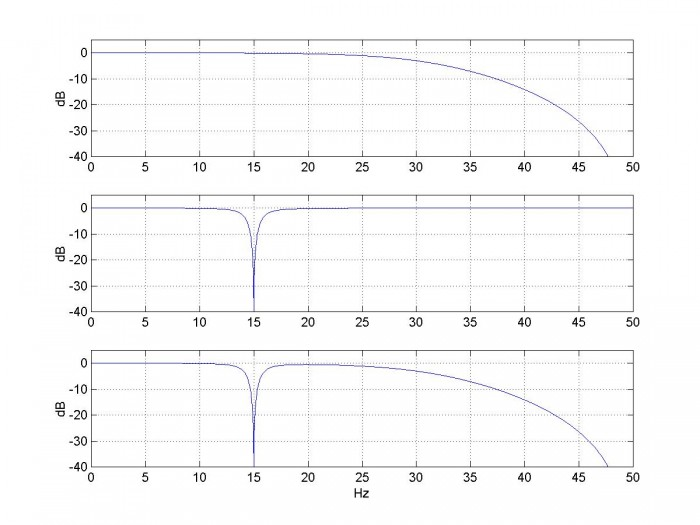# Coefficients of Cascaded Discrete-Time Systems

March 4, 2018

In this article, we’ll show how to compute the coefficients that result when you cascade discrete-time systems.  With the coefficients in hand, it’s then easy to compute the time or frequency response.  The computation presented here can also be used to find coefficients of mixed discrete-time and continuous-time systems, by using a discrete time model of the continuous-time portion .

Consider the cascade of two systems, H1(z) and H2(z), where

$$H_1(z)=K_1\frac{b_0+b_1z^{-1}+...+b_Nz^{-N}}{1+a_1z^{-1}+...+a_Nz^{-N}} = K_1\frac{b_0z^N+b_1z^{N-1}+...+b_N}{z^N+a_1z^{N-1}+...+a_N }$$

$$H_2(z)=K_2\frac{d_0+d_1z^{-1}+...+d_Mz^{-M}}{1+c_1z^{-1}+...+c_Mz^{-M}} = K_2\frac{d_0z^M+d_1z^{M-1}+...+d_M}{z^M+c_1z^{M-1}+...+c_M }$$

H1(z) and H2(z) are assumed to have the same sample rate.  H(z) = H1(z)H2(z) can be found by multiplying-out the numerator and denominator polynomials of H1 and H2.  This can be done by hand, but it turns out there is a rule for multiplying polynomials .  The rule is as follows:  Given two polynomials with coefficients b = [b0 b1 … bn] and d = [d0 d1 … dn], the coefficients of the product of the polynomials are given by the convolution of b and d.Thus H(z) = H1(z)H2(z) has coefficients:

numerator coeffs = b  d            (1)

denominator coeffs = a  c        (2)

where ⊛ indicates convolution.  The overall gain constant is K1K2.  For FIR systems, the denominator is unity, so the coefficients of the cascaded systems are b⊛d (which is perhaps no surprise).

## Example

Here we’ll cascade 2nd-order lowpass and band-reject IIR filters with transfer functions H1(z) and H2(z) as follows:

$$H_1(z)=K_1\frac{b_0+b_1z^{-1}+b_2z^{-2}}{1+a_1z^{-1}+a_2z^{-2}}$$

where H1(z) is lowpass with

    a= [1 .3695 .1958]
b= [1 2 1]
K1= .3913


$$H_2(z)=K_2\frac{d_0+d_1z^{-1}+d_2z^{-2}}{1+c_1z^{-1}+c_2z^{-2}}$$

where H2(z) is band-reject with

    c= [1 -1.1074 .8841]
d= [1 -1.1756 1]
K2= .942


The frequency responses of the two filters are computed using Matlab as follows:

    fs= 100;                       % Hz sample frequency
[h1,f]= freqz(K1*b,a,2048,fs);
H1= 20*log10(abs(h1));         % dB magnitude response
[h2,f]= freqz(K2*d,c,2048,fs);
H2= 20*log10(abs(h2));         % dB magnitude response


The responses are plotted in Figure 1.  The cascade coefficients are computed using Equations 1 and 2:

    bb= conv(b,d)
aa= conv(a,c)
K= K1*K2


This gives coefficients:

    aa =   1.0000   -0.7379    0.6707    0.1098    0.1731
bb =   1.0000    0.8244   -0.3512    0.8244    1.0000
K =    0.3686


The length of aa is 5, so the cascade transfer function is 4th order.  The transfer function is:

$$H(z)=K\frac{bb_0+bb_1z^{-1}+bb_2z^{-2}+bb_3z^{-3}+bb_4z^{-4}}{1+aa_1z^{-1}+aa_2z^{-2}+aa_3z^{-3}+aa_4z^{-4}}$$

(I apologize for the ungainly notation).  The frequency response is:

    [h,f]= freqz(K*bb,aa,2048,fs);
H= 20*log10(abs(h));           % dB magnitude response


The cascaded response is shown in the lower plot of Figure 1.

In case you are interested, the lowpass and band-reject coefficients were calculated using the following Matlab code:

    fs= 100;
fc= 30;
[b,a]= butter(2,2*fc/fs)         % lowpass coeffs
f0= 15;
fU= 16;
[d,c]= br_synth1(1,f0,fU,fs)     % band-reject coeffs


Here, br_synth1 is a Matlab function developed in an earlier post .Figure 1.  Magnitude response of cascaded lowpass and Band-reject filters.

top:  Lowpass response |H1|    middle:  Band-reject response |H2|    bottom:  cascade response

Neil Robertson            March, 2018

## Appendix      Convolution Formulas

The output y[n] of an FIR filter is the discrete convolution of its coefficients b with an input x[n].  We call each of these terms a “sequence”.  Discrete convolution is defined as :

$$y[n]=\sum_{k=-\infty}^{\infty}b[k]\cdot x[n-k]$$

$$=\sum_{k=0}^N b[k]\cdot x[n-k]$$

where N= length(b)-1 and indices of x that are negative or greater than length(x)-1 are ignored.

Cascading two FIR filters uses the same convolution formula.  However, the two sequences are the coefficients of the filters.  When multiplying polynomials, the two sequences are the polynomial coefficients.  In these cases, since we are not dealing with an input signal x, we replace x with another symbol.  Thus the convolution of two discrete sequences b and d is:

$$y[n]=\sum_{k=0}^N b[k]\cdot d[n-k]$$

Where, again, N= length(b)-1.  Convolution is commutative, so we can also write the above equation as:

$$y[n]=\sum_{k=0}^M d[k]\cdot b[n-k]$$

where M= length(d)-1.

## References

1.Robertson, Neil, https://www.dsprelated.com/showarticle/1055.php

2.Smith, Julius O., Mathematics of the Discrete Fourier Transform (DFT), https://www.dsprelated.com/freebooks/mdft/Polynomial_Multiplication.html

3.Robertson, Neil, https://www.dsprelated.com/showarticle/1131.php

4.Lyons, Richard G., Understanding Digital Signal Processing, 2nd Ed., Prentice Hall, 2004, section 5.9.1.

To post reply to a comment, click on the 'reply' button attached to each comment. To post a new comment (not a reply to a comment) check out the 'Write a Comment' tab at the top of the comments.